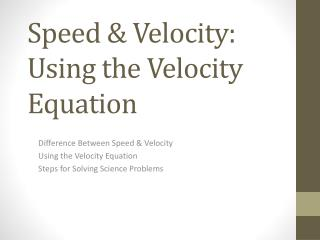Download PresentationSpeed & Velocity: Using the Velocity Equation

Speed & Velocity: Using the Velocity Equation - PowerPoint PPT Presentation

Speed & Velocity: Using the Velocity Equation. Difference Between Speed & Velocity Using the Velocity Equation Steps for Solving Science Problems. I. Speed and Velocity. Speed is a measure of how fast an object is movingI am the owner, or an agent authorized to act on behalf of the owner, of the copyrighted work described.
Download PresentationSpeed & Velocity: Using the Velocity Equation

Download Policy: Content on the Website is provided to you AS IS for your information and personal use and may not be sold / licensed / shared on other websites without getting consent from its author.While downloading, if for some reason you are not able to download a presentation, the publisher may have deleted the file from their server.

- - - - - - - - - - - - - - - - - - - - - - - - - - E N D - - - - - - - - - - - - - - - - - - - - - - - - - -
Presentation Transcript
1. Speed & Velocity: Using the Velocity Equation Difference Between Speed & Velocity Using the Velocity Equation Steps for Solving Science Problems

2. I. Speed and Velocity • Speed is a measure of how fast an object is moving • Words like fast and slow describe speed but aren’t very descriptive. After all, a fast ant is nowhere as fast as a fast car. • 50 mi/hr is a better way of defining speed • This tells you how far you travel in one hour.

3. I. Speed and Velocity • Velocity tells you the speed and direction of an object • 65 mi/hr due south is a velocity because it tells you the direction of travel as well as the speed. • We will be using positive and negative to describe the direction • Positive velocities are moving forward • Negative Velocities are moving backward

4. Comprehension Check • Identify each of the following as either a speed or a velocity. • We drove north at 28.543 mi/hr. • Running hard, I can run 0.2 mi/min. • A skydiver fell at a rate of 124 mi/hr toward the ground. • “Kinetic energy penetrator ammunition” fired from tank guns travel at more than 1 mi/s. Velocity Speed Velocity Speed

5. Comprehension Check • Describe the speed and direction of each of the objects below. • Shannon was running at a positive 9m/s. • Shannon traveled 9m every second in the forward direction. • Kari walked at -1.5m/s through the hall. • Kari walked 1.5m backwards every second. • Michael was riding in a car that was traveling -10mi/hr. • Michael’s car was moving 10 miles backward every hour.

6. II. Using the Velocity Equation • Units of Speed and Velocity • mi/hr, ft/s, m/s, km/h, etc • All follow the pattern of distance/time • The velocity equation can be stated as • If you know any two of the variables, you can calculate the third variable using the equation. or

7. III. Steps for Solving Science Problems • Step 1: Read the problem carefully, and identify what you are asked to find. • Step 2: Write down the information you are given. • Step 3: Write down any equations you know that are related to the info given. • Step 4: Pick an equation to start with and solve it for the variable you are looking for. • Step 5: Plug in the numbers and calculate the answer.

8. d= t= v= Example 1 – Calculating Velocity • If a car travels 309 miles in 6.8 hours, what is the speed of the car in miles per hour? Step 1 & 2: Identify what you are looking for & write down what you know Step 3: Write down the velocity equation Step 4: Solve for the unknown Step 5: Plug in numbers and calculate 309mi 6.8hrs ?

9. d= t= v= Example 2 – Calculating Distance • Light travels at the astonishing rate of 11,176,943 mi/min. If it takes light an average of 8.3 minutes to reach Earth from the sun, what is the average distance, in miles, between the Earth and Sun? Step 1 & 2: Identify what you are looking for & write down what you know Step 3: Write down the velocity equation Step 4: Solve for the unknown Step 5: Plug in numbers and calculate ? 8.3min 11,176,943mi/min d=vt=(11,176,943mi/min)8.3min d=92,768,626 mi or 93,000,000mi

10. d= v v t= v= Example 3 – Calculating Time • The muzzle velocity of a paint ball gun can reach speeds of 152 m/s. If the paintball maintains that speed, how many seconds will it take to hit a person standing 33 meters away? Step 1 & 2: Identify what you are looking for & write down what you know Step 3: Write down the velocity equation Step 4: Solve for the unknown Step 5: Plug in numbers and calculate 33m d=vt ? 152m/s